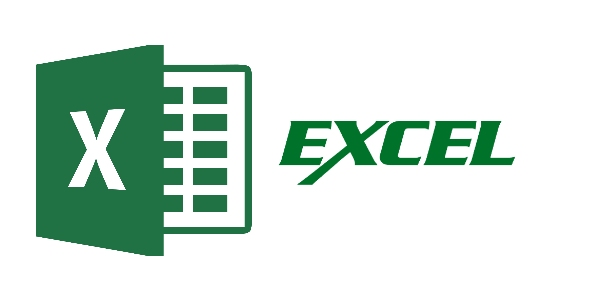# Microsoft Excel Vocabulary And Functions! Trivia Quiz

10 Questions | Total Attempts: 708SettingsMicrosoft Excel is a spreadsheet application produced by Microsoft that allows users to organize and calculate data with formulas. It's used for performing the basic calculation, graphing tools, creating pivot tables and macros. This quiz has been created to test your knowledge and skills about spreadsheet vocabulary, functions and formulas. So, let's try out the quiz. All the best!

Related Topics
• 1.
A cell formula that stays the same when copied
• A.

Absolute reference

• B.

Relative reference

• C.

Active cell

• D.

Formula

• 2.
Saved workbook or worksheet
• A.

Document

• B.

File

• C.

Grid

• D.

Graph

• 3.
The vertical divisions of a spreadsheet
• A.

Cell

• B.

Row

• C.

Column

• 4.
A tab that identifies a worksheet
• A.

Sheet tab

• B.

Sheet

• C.

Formula

• D.

Active cell

• 5.
Name of boxes (cells) in a spreadsheet
• A.

Graph

• B.

• C.

• D.

Worksheet

• 6.
What tool is used to split the window into different parts of the same worksheet?
• A.

The title bar

• B.

The scroll bar

• C.

The toolbar

• D.

The split box

• 7.
What key is used to select multiple ranges of cells?
• A.

Shift

• B.

CTRL

• C.

Alt

• D.

AltCar or AltGr

• 8.
What tool helps you to optimize the results of a model?
• A.

Scenario manager

• B.

Solver

• C.

Target value

• D.

None of the above

• 9.
What function offers you the possibility to view different results depending on the entered condition?
• A.

=If()

• B.

=Sum()

• C.

=SumIf()

• D.

=TrueFalse()

• 10.
What function is used to make sure that all conditions are true before showing a TRUE answer?
• A.

=AND()

• B.

=OR()

• C.

=Vlookup()

• D.

=If()